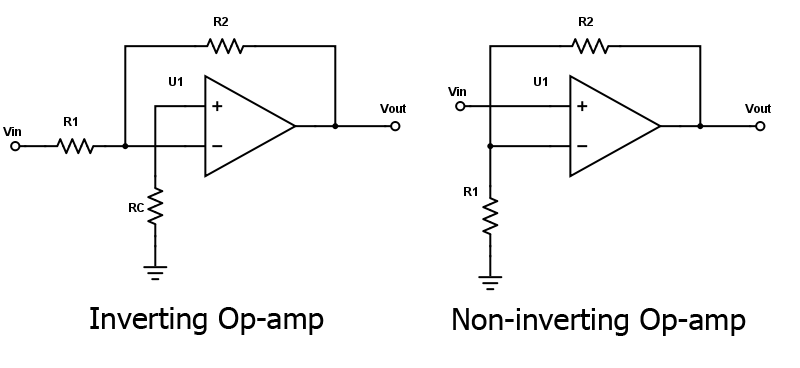# Inverting Op-Amp Resistor Calculator

## This calculator determines the bias and feedback of resistors for an inverting op-amp.

V

V
V
V
V

### Overview

An inverting operational amplifier (op-amp) amplifies the input signal while inverting its polarity. This calculator is designed to compute for the resistors R2, R3 and R4 given the other parameters. The resulting values are in kilo-ohms (kΩ).### Equations

$$R_{2} = -A R_{1}$$

$$R_{3} = R_{1}$$

$$R_{4}= R_{3}\left[\frac{(R_{1}+R_{2})V_{2}-V_{out2}}{V_{out2}R_{1}}\right]$$

$$V_{out1}= A V_{1}$$

$$V_{out2}= V_{out}-V_{out1}$$

### Applications

The operational amplifier is a staple in electronics design as it can be found in hundreds of applications. The most common uses of the op-amp includes amplification, as buffer, as controlled source and in active filters.

#### Amplifier

The op-amp can be configured as a non-inverting amplifier or as an inverting amplifier. The circuits for these two amplifier configuration is shown below:The gain of the inverting op-amp can be calculated using the formula:

$$A = -\frac{R_{2}}{R_{1}}$$,

while the gain of the non-inverting op-amp is given as:

$$A = 1 + \frac{R_{2}}{R_{1}}$$

Any of these amplifier configurations can be cascaded for greater gain. Cascading two inverting op-amps can also return the signal to its normal polarity.